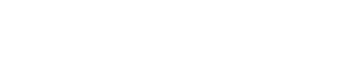# IBPS PO 2017 Practice Test – Quant Day 11

Hello Aspirants.

Welcome to Online Reasoning Section with the explanation in AffairsCloud.com. Here we are creating Best question samples for IBPS PO 2017. We have included Some questions that are repeatedly asked in bank exams !!!

IBPS PO 2017: Reasoning Test– 6 PM Every Day
IBPS PO 2017: Quants Test– 7 PM Every Day

1. Dinesh Spent 80% of his income in the year 2015. At the end of year he divided the rest of money in the ratio of 9:11 for his Daughter and Son and deposited in two different banks for 2 years which offered 6% and & 7% per annum to Son and Daughter respectively. If the total interest earned from both the Banks by Dinesh is 21672 then Dinesh income in year 2015 is?
A. 7.2 Lakhs
B. 7.7 Lakhs
C. 8.4 Lakhs
D. 8.8 Lakhs
E. None
C. 8.4 Lakhs
Solution:
21672 = X ( 2*7/100 * 20/100 * 9/20 + 6*2 /100 * 20/100 * 11/20 )
X = 8.4 Lakhs

2. Population of Village in 2013 is 56732. Ratio of Population in that village in 2014 to 2015 is 5:6. Then percentage increase in population from 2013 to 2014 is
A. 5%
B. 10%
C. 15%
D. 20%
E. Cannot be determined
E. Cannot be determined
Solution:
From the above given data it cannot be determined

3. 200 workers are employed to work . They were paid Rs. 23616 in all. Ratio of wages of one Male to One female is 3:2. Ratio of Males to females is 23:27. Then the difference between total wages of Male and Female workers is?
A. 2460
B. 2880
C. 3120
D. 3920
E. None
B. 2880
Solution:
No of Males = 200*23/50 = 92
No of Females = 200*27/50 = 108
Total Money paid = 23616 = (92*x + 108 * y)
X:Y = 3:2
23616 = (92*3/2*y + 108 *y)
Y = 96
X = 144
Difference = 92* 144 – 108 * 96 = 2880

4. Sekhar consumption of Rice and Wheat in month of July is 50 & 45 Kg respectively. Price of Rice is 20% more than price of Wheat. Total price of Rice and Wheat is Rs 2100. In month of August Price of Wheat increased by 20% and Rice is maintained at the same price. Then what amount of consumption of Rice in Kgs has to reduced to buy both Rice and Wheat at Rs 2100?
A. 5 kg
B. 7.5 kg
C. 10 kg
D. 10.5 kg
E. None
B. 7.5 kg
Solution:
2100 = (50*R*120/100 + 45*R)
R = 20
In August
Wheat price = 24
(50-x) * 24 + 24 *45 = 2100
X = 7.5

5. Rajesh 25 defective Mobiles for Rs 60,000. He repaired the mobiles and sold each at Rs 3200. He got profit of Rs 600 on each Mobile. How much did he spend on repairs?
A. Rs. 2000
B. Rs. 4000
C. Rs. 5000
D. Rs. 8000
E. None
C. Rs. 5000
Solution:
CP of one item = 60,000/25 =2400
3200-2400 =800
But repairs cost is 600
800-600 = 200
Total repair cost = 200*25 = 5000

Q(6-10) The Bar Graph shows different items sold by different shopkeepers in the year 2016. Table shows CP of single item.1. If all the Shop keepers sold all items with the in bulk to a certain school each at Rs. 6000 then which shopkeeper would make maximum profit?
A. Shop A
B. Shop B
C. Shop C
D. Shop D
E. Shop E
C. Shop C
Solution:
Shop A: 72*13+ 73*14+67*15+66*16+70*17 = 5209
Shop B: 75*13+64*14+74*15+71*16+72*17 =5341
Shop C: 72*13+71*14+63*15+67*16+69*17 = 5120
Shop D: 64*13+69*14+68*15+75*16+66*17 = 5140
Shop E: 72*13+64*14+74*15+69*16+17*68 = 5267
Profit = 6000 – 5209 = 791
Profit = 6000 – 5341 = 659
Profit = 6000 – 5120 = 880
Profit = 6000 – 5140 = 860
Profit = 6000 – 5267 = 733

2. What is the average no of Pencils sold by all shop keepers?
A. 69
B. 70
C. 71
D. 72
E. 73
D. 72
Solution:
72+75+72+64+77 = 360 average = 360/5 = 72

3. What is the difference between the total amount spent by all shopkeepers on Books and Pencils?
A. 1397
B. 1497
C. 1553
D. 1185
E. 1623
D. 1185
Solution:
70*17+72*17+69*17+66*17+68*17 = 5865
72*13+75*13+72*13+64*13+77*13 = 4680
Difference = 5865 – 4680 = 1185

4. The difference between cost of total items sold by Shop Keeper C & D in rupees is?
A. Rs. 10
B. Rs. 15
C. Rs. 20
D. Rs. 25
E. None
C. Rs. 20
Solution:
Shop C: 72*13+71*14+63*15+67*16+69*17 = 5120
Shop D: 64*13+69*14+68*15+75*16+66*17 = 5140
Difference = 5140 -5120 = 20

5. If shop keeper D sold each Pencils, Erasers, Pens, Sharpners and Books at 10%, 5%, 15%, 10% and 20% profits respectively then his total profit would be?
A. Rs. 619
B. Rs. 629
C. Rs. 639
D. Rs. 649
E. None